Home | | Maths 6th Std | Measures of Time

# Measures of Time

The procedure of measuring time has undergone several changes from ancient period.

Measures of Time

* How long do you take to run 100 metres?

* How long do you take to walk one kilometer?

* What is the time taken for a cup of rice to be cooked?

* What is the cultivation period of groundnut?

These questions will help us to find the importance of time in our day-to-day life.

Now let us discuss the development of measures of time.

The procedure of measuring time has undergone several changes from ancient period. Initially, a stick in the sand was used to measure time by measuring the length of the shadow of that stick. Then horizontal and vertical plates were used as sundials to measure time between sunrise and sunset, that is in the day time. Firing of knotted ropes was used to measure time in the darkness. The approximate time taken for the fire to travel from one knot to other formed the part of night. In later days, a day was divided into 24 equal parts (hours) of which 12 hours were for daytime and 12 hours were for night time approximately.

Time taken by the Earth to complete one full rotation around the Sun is known as the Solar Year. It was divided into 12 equal parts which is known as the Solar month. The duration between two full moons is known as the lunar month and 12 lunar months are known as lunar year. But we follow solar year and month.

Various clocks had been designed and used to measure time from different parts of world, like water clock, sun dial candle clock, sand clock, rope clock, etc . Have you seen those clocks? Look at the clocks shown below.Study of devices that are measuring the time is called ‘HOROLOGY’.

Nowadays, we use pendulum clock, digital clock, quart clock, atomic clock to find time accurately.

Our Tamil people were experts in the Astronomical science. The Tholkappiam deals the pozhuthu (time). They divide a day into six major divisions, together called “Sirupozhuthu (சிறுபொழுது)” . A year into six major divisons, together called “Perumpozhuthu (பெரும்பொழுது)”

1 Nahigai = 24 min; 1 hour = 2.5 nazhigai = 1 Orai; 1 day = 24 hours = 60 nazhigai

Unit of Time: Today we are measuring time accurately. The units of time are second, minute, hour, day, week, month, year, etc. They are interrelated.

Recap:

1. Read and write the time in the appropriate place.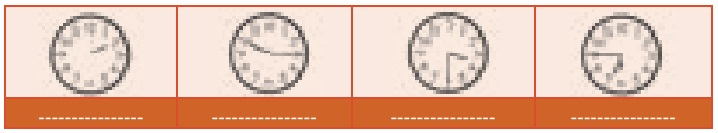Practise to say time in two ways:-Practise to say the time using "past"Practise to say the time using "To"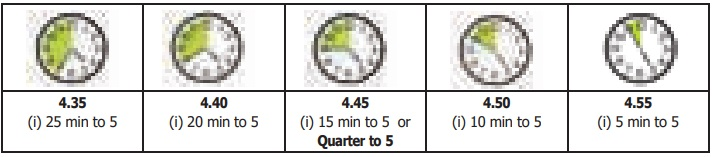Try these

Say the following time in two ways:

a) 9.20

20 minutes past 9

40 minutes to 10

b) 4.50

50 minutes past 4

10 minutes to 5

c) 5.15

15 minutes past 5

45 minutes to 6

d) 6.45

45 minutes past 6

15 minutes to 7

e) 11.30

30 minutes past 11

30 minutes to 12

2. Conversion of Time

Calculation of time to the nearest seconds is very essential in some situations like launching, rocket, running race, arrival and departure. So, we need to know the conversion of time.

Let us remember the time related chart as follows:

Example 12: A farmer ploughed the paddy field for 3 hours 35 minutes. How many minutes did he plough?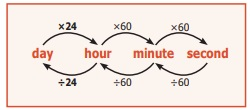Solution:

Time for which the farmer ploughed the paddy field = 3 hours and 35 minutes

= 3 × 60 minutes + 35 minutes

= 180 minutes + 35 minutes

= 215 minutes

Examples 13: A handloom weaver takes 6 hours 20 minutes 30 seconds and 5 hours 50 minutes 45 seconds to weave two silk sarees. What is the total time to weave the two silk sarees?

Solution:Example 14:

A satellite is placed in its orbit in 7 hours 16 minutes 20 seconds. Calculate it in seconds.

Solution: The satellite reaches its orbit in 7 hours + 16 minutes + 20 seconds

= (7 × 60 × 60) seconds + (16 × 60) seconds +20 seconds

= 25200 seconds + 960 seconds + 20 seconds

= 26,180 seconds

The satellite reaches its orbit in 26,180 seconds

Example 15:

Two cyclists took 5 hours 35 minutes 10 seconds and 8 hours to cover the same distance. Find the difference in time taken by them

Solution:Try these

Convert the following:

i) 4 hours = 240 minutes

ii) 240 minutes = 4 hours

iii) 30 minutes = 1800 seconds

iv) 3600 seconds = 1 hours

v) 2 hours = 7200 seconds

3. Ordinary Time or the 12-Hour Format

The 12 hour clock has antemeridien (a.m) and postmeridien (p.m) because the number of hours in a day is divided into day and night. In the clock, exactly 12.00 at night is called midnight; and exactly 12.00 at day is called noon.

a.m (antemeridien) denotes the time that is after 12:00 midnight and before 12:00 noon. p.m (postmeridien) denotes the time that is after 12:00 noon and before 12:00 midnight

Example:

* Morning 5 o’clock is denoted as 5.00 a.m

* Evening 5 o’clock is denoted as 5.00 p.m

* In 3.20 a.m., the point does not mean the usual decimal point.4. Railway Time or the 24-Hour Format

Generally, we use 12 hour clock but Railways, Airways, Defence forces and Television networks use 24 hour clock to avoid morning or evening confusions. When you are in a railway station, you can hear the announcement and see the use of hours instead of a.m. and p.m, because they follow the 24 hour format. Therefore, there is no need to say morning and evening in their time. Railway time is usually denoted in 4 digits. The first two digits shows the hours and the last two digits shows the minutes. For example, 5 p.m is denoted as 17:00 hours.Example: 7 o' clock morning = 07:00 hours

1 o' clock evening = 13:00 hours (12+1 hour)

i.e., after 12 noon they count continuously up to 24 hours.

12 midnight is written as 00:00 hours or 24:00 hours.

12 noon is written as 12:00 hours5. Conversion of Time Formats

Let us observe the clock. Remember the following points while converting from one type of time to another type

* To convert 12 hour time to 24 hour time, simply change 12 hours as 00:00 hours between 12.00 midnight and 01.00 a.m there is no change upto 01.00 p.m. Add 12:00 hours to any hour from 01.00 p.m.* To convert 24 hour time to 12 hour time simply change 00:00 hours as 12 hours between 00:00 hours and 01:00 hour. There is no change upto 13:00 hours. Subtract 12:00 hours from any hours from 13:00 hours. Minutes will not change in both the formats

Convert into the 12 hour format: (Ordinary time)Convert into the 24 hour format (Railway time )Try these

Convert the 12 hour format into the 24 hour format and vice versa

10.40 a.m = 10:40 hours

11 a.m = 11:00 hours

1.15 a.m = 01 : 15 hours

5 a.m =  05 : 00 hours

16:20 hours =  4 : 20 p.m. a.m/p.m

00:40 hours =  12 : 40 a.m.   a.m/p.m

1 p.m = 13:00 hours

11.15 p.m = 23:15 hours

3 p.m =  15 : 00 hours

12 midnight =  00 : 00 hours

12:25 hours =  12 : 25 a.m  a.m/p.m

4:10 hours =  4 : 10 a.m. a.m/p.m

6. Duration between the two given time instances:

Example16:

Find the duration between 6 a.m and 4 p.m

Solution :

Method-1

Conversion of 6 a.m to Railway time = 06:00 hours

Conversion of 4 p.m to Railway time = (4+12) hours = 16:00 hours

Time duration between 6 a.m and 4 p.m = The difference between 16 hours and 6 hours = 16 hours − 6 hours = 10 hours

Method-2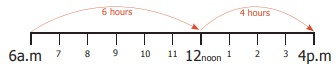= 6 hours + 4 hours = 10 hours

Example 17:

The arrival and departure timings of the Chennai − Trichy Express are given below.(i) At what time does the train depart from Chennai Egmore to arrive at Trichy?

The train departs from Egmore at 20:30 hours to arrive Trichy at 4:30 hours.

(ii) How long does it halt at Villupuram ?

It halts at Villupuram for 10 minutes.

(iii). How many halts are there in between Chennai and Trichy?

There are 3 halts (1) Chengalpattu (2) Villupuram (3) Virudhachalam

(iv). Find the total journey time of the train from Chennai to Trichy.

Hint: If the journey crosses the midnight, calculate the time duration from starting hours to midnight, then from midnight to arrival time.Duration from midnight to arrival = 4 hours 30 minutes

Total journey time = Duration up to midnight + Duration from midnight to arrival

= 3 hours 30 minutes + 4 hours 30 minutes

= 7 hours 60 minutes = 7 hours + 1 hour = 8 hours

Example 18:

The clock is set at 7 a.m. If the clock slows down two minutes every hour, find the time shown by the clock at 6 p.m.

Solution : Time slowed down for 1 hour = 2 minutes

Time slowed down for 11 hours = 11 × 2 = 22 minutes

So, at 6 p.m the clock slows down by 22 minutes. That means the clock shows 5 hours 38 minutes at 6 p.m.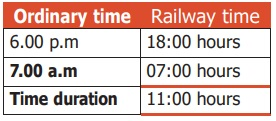7. Year

A year is the time taken by the Earth to make one revolution around the Sun. A year has 12 months or 365 days. Each month is divided into weeks. A month has 4 weeks and a few more days. A week is of 7 days. A month has 30 days / 31 days except February. February has 28 or 29 days.

Leap Year

We know that the Earth revolves around the Sun as well as rotates to itself. The Earth takes 365 days 6 hours to make a complete revolution around the sun. We take 365 days as one year. To adjust 6 hours each year, we add one day to every fourth year (4 years 6 hours = 24 hours = 1 day). Every 4th year has 365 +1 day = 366 days and one day is added to the month of February. Therefore a year which has 366 days is called a Leap Year. In a Leap Year the month of February has 29 days. Every year you are celebrating birthday. If a person’s birthday falls on 29th February, he/she has to celebrate the birthday once in 4 years only.How can we identify a leap year?

I. Generally a year which is divisible by 4 is considered as a leap year.

Examples:

1. 2016 is a leap year, because 2016 is exactly divisible by 4

2. 2018 is not divisible by 4 and it leaves remainder. So it is not a leap year.II. In centuries:

Years which are multiples of 100 are centuries, such as 1100, 1200, 1300….1900, 2000, 2100 ….etc. The century which is divisible by 400 is a leap year.

Think

Why do we divide the centuries by 400 to find whether it is leap year or not?

Examples:

1. 1200 is divisible by 400; and so it is a leap year.

2. 1700 is not divisible by 400. and so it is not a leap year.

Example 19:

If  Wednesday falls on 20th of December 2017.  what is the day on 8th  june 2018? Also say the number of days between these two dates.170 day ÷ 7 (why? )

170 days = 24 weeks +2 days

Required day is the second day after Wednesday.

Therefore 8th of june is Friday.

Example 20:

Mala’s date of birth is 20-11-1999. What is her age on 05-10-2018?

Solution:

Convert in the format : YYYY/MM/DDMala's age : 18 yrs 10 months 15 days

Activity

1. Collect some famous personalities whose birthday falls on 29th February.

2. Collect the interesting facts about Big Ben clock in London.

Try these

1. Check whether the following years are Ordinary or Leap Year ?

1994; 1985; 2000; 2007; 2010; 2100

1994

94 / 4  remainder 2

1994 is an ordinary year.

1985

85 / 4  remainder 1

1985 is an ordinary year.

2000

2000  / 400 remainder 0

2000 is a leap year.

2007

07 / 4 remainder 3

2007 is an ordinary year.

2010

10 / 4 remainder 2

2010 is an ordinary year.

2100

2100 / 400    remainder 1

2100 is an ordinary year.

2. How many days are there from 1st April to 30th June?Month  : Number of days

April : 30

May : 31

June : 29

90

Total 90 days

A Jubilee is a particular anniversary of an event.

Silver Jubliee : 25th anniversary

Ruby Jubilee : 40th anniversary

Golden Jubilee : 50th anniversary

Diamond Jubilee : 60th anniversary

Sapphire Jubilee : 65th anniversary

Platinum Jubilee : 70th anniversary

* 10 years = 1 decade

* 100 years = 1 century

* 1000 years = 1 millennium

* 21st century 2001 – 2100, we are in this century

* 3rd millennium − 2001 − 3000 yrs, we are in this millennium

ICT CORNER

Measurements

Expected OutcomeStep 1

Open the Browser and type the URL Link given below (or) Scan the QR Code. GeoGebra work sheet named “Measurement Unit convertor” will open. The work sheet contains three activities.

1. Length convertor and 2.Weight convertor and 3. Convertor for all measurements

In the first activity move the sliders to change the value of kilometre, metre and centimetre and check the conversion.

Step 2

In the second activity move the sliders to change the value of Kilogram, Gram and Milligram and check the conversion.